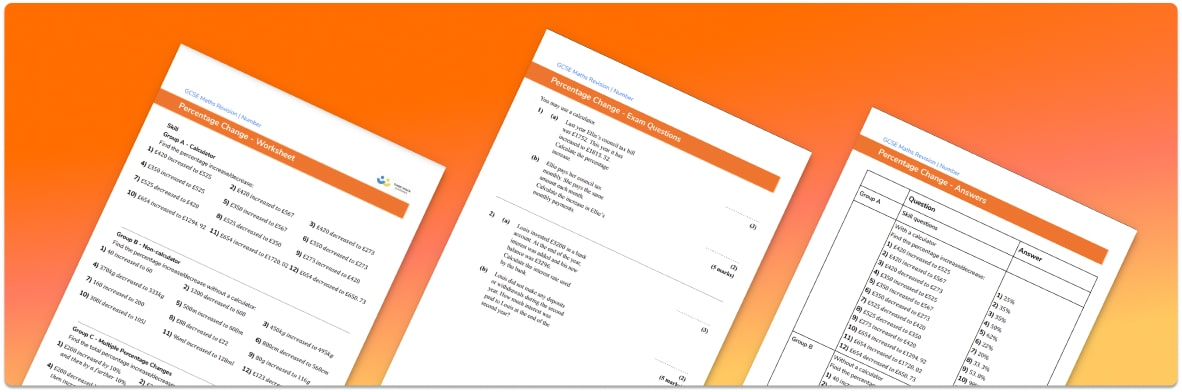# Percentage Change Worksheet• Section 1 of the percentage change worksheet contains 36 skills-based percentage change questions, in 3 groups to support differentiation
• Section 2 contains 4 applied percentage change questions with a mix of word problems and deeper problem solving questions
• Section 3 contains 4 foundation and higher level GCSE exam style questions on  percentage change
• Answers and a mark scheme for all percentage change practice questions are provided
• Questions follow variation theory with plenty of opportunities for students to work independently at their own level
• All maths resources created by fully qualified expert secondary maths teachers

Suitable for GCSE maths revision for AQA, OCR and Edexcel exam boards

• This field is for validation purposes and should be left unchanged.

You can unsubscribe at any time (each email we send will contain an easy way to unsubscribe). To find out more about how we use your data, see our privacy policy.

### Percentage change at a glance

Percentage change is the overall term for percentage increase and decrease. When something is increased by a given percentage, we can use mental calculation strategies or the percentage multiplier method to calculate its new value. For example, if something costs £35 and is increased by 20%, to find the new price we could either find 20% of £35 and add this to the original amount or work out 1.20 35.

If we were required instead to decrease by 20%, we could use subtraction from the original value, or use the percentage multiplier 0.8 (a 20% reduction means we are finding 80% of the original amount).

Sometimes, students are required to find the percentage change – for example, given the original and new values, calculate percentage profit or percentage loss. Extending this topic further, students study the reverse process – given the new number and the percentage change, work backwards to find the original number.

Looking forward, students can then progress to additional number worksheets, for example a rounding to decimal places worksheet or a standard form worksheet.For more teaching and learning support on Number our GCSE maths lessons provide step by step guides for all GCSE maths concepts.

## Do you have KS4 students who need more focused attention to succeed at GCSE?There will be students in your class who require individual attention to help them succeed in their maths GCSEs. In a class of 30, it’s not always easy to provide.

Help your students feel confident with exam-style questions and the strategies they’ll need to answer them correctly with our dedicated GCSE maths revision programme.

Lessons are selected to provide support where each student needs it most, and specially-trained GCSE maths tutors adapt the pitch and pace of each lesson. This ensures a personalised revision programme that raises grades and boosts confidence.

Find out more